Examples

Chapter 11 Class 12 Three Dimensional Geometry
Serial order wise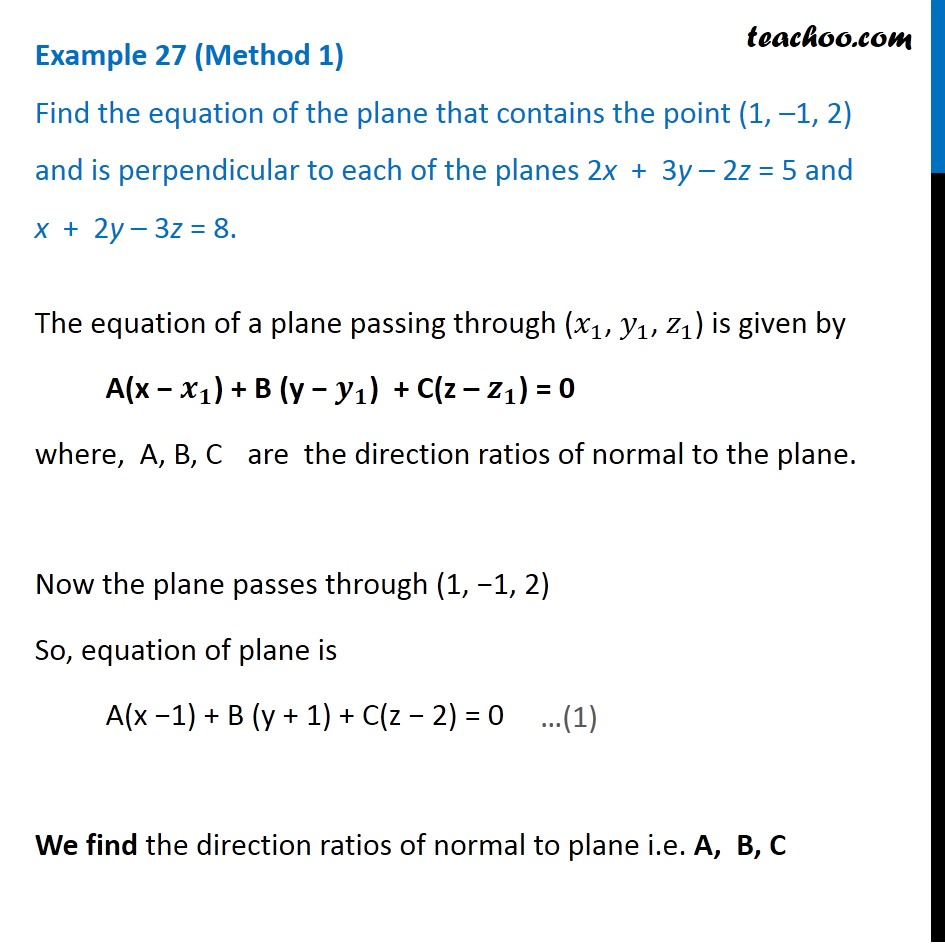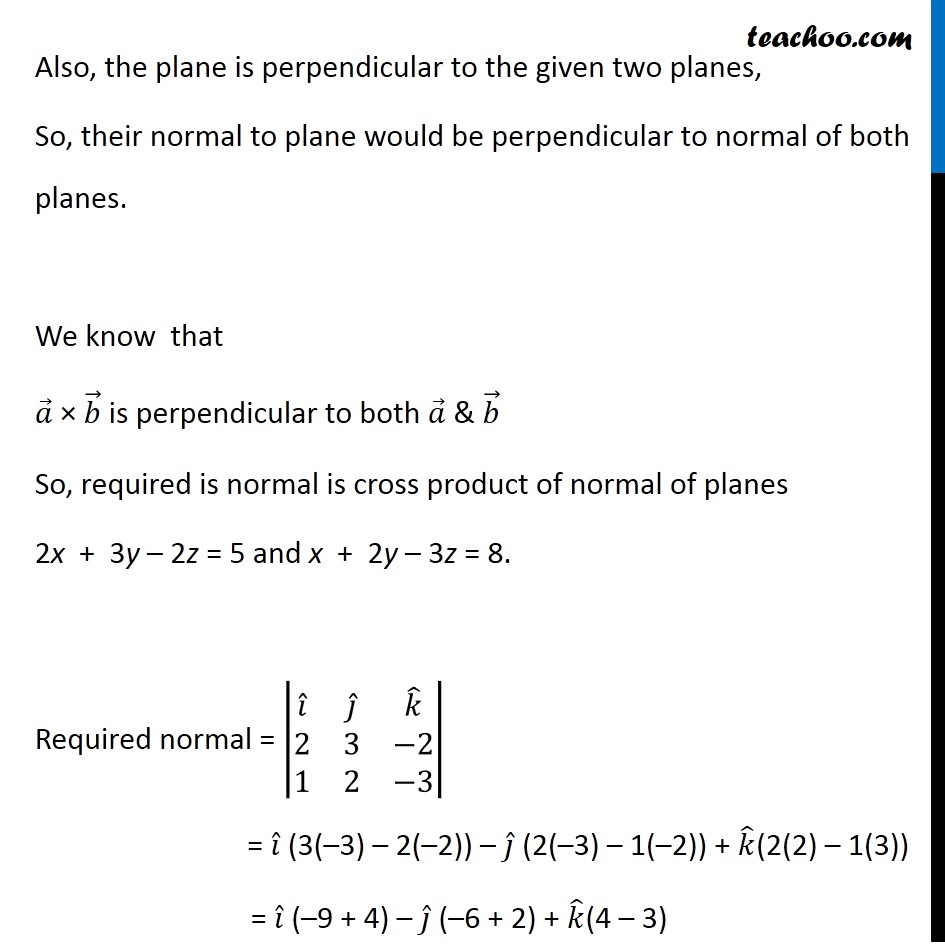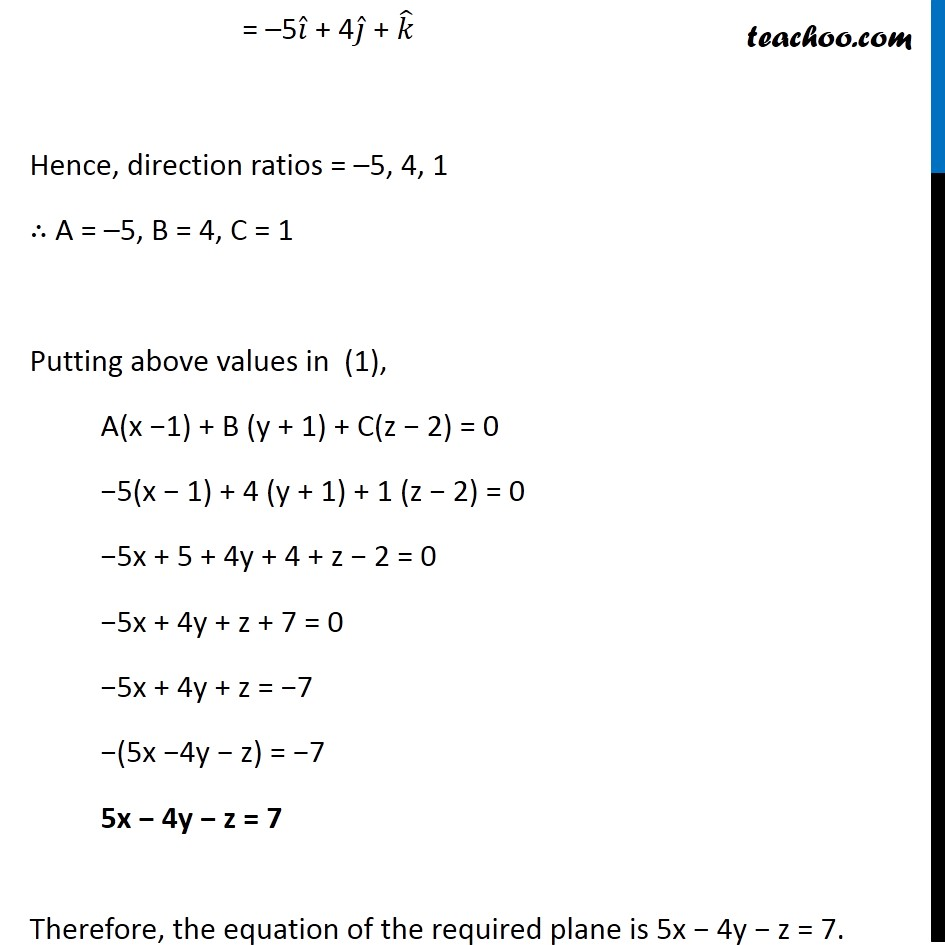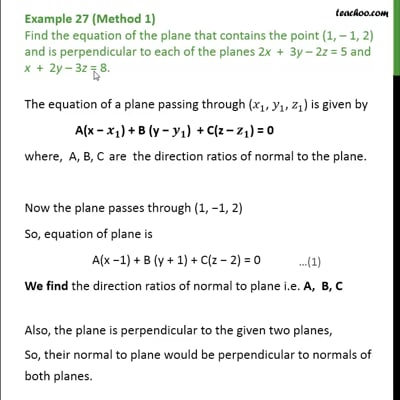This video is only available for Teachoo black users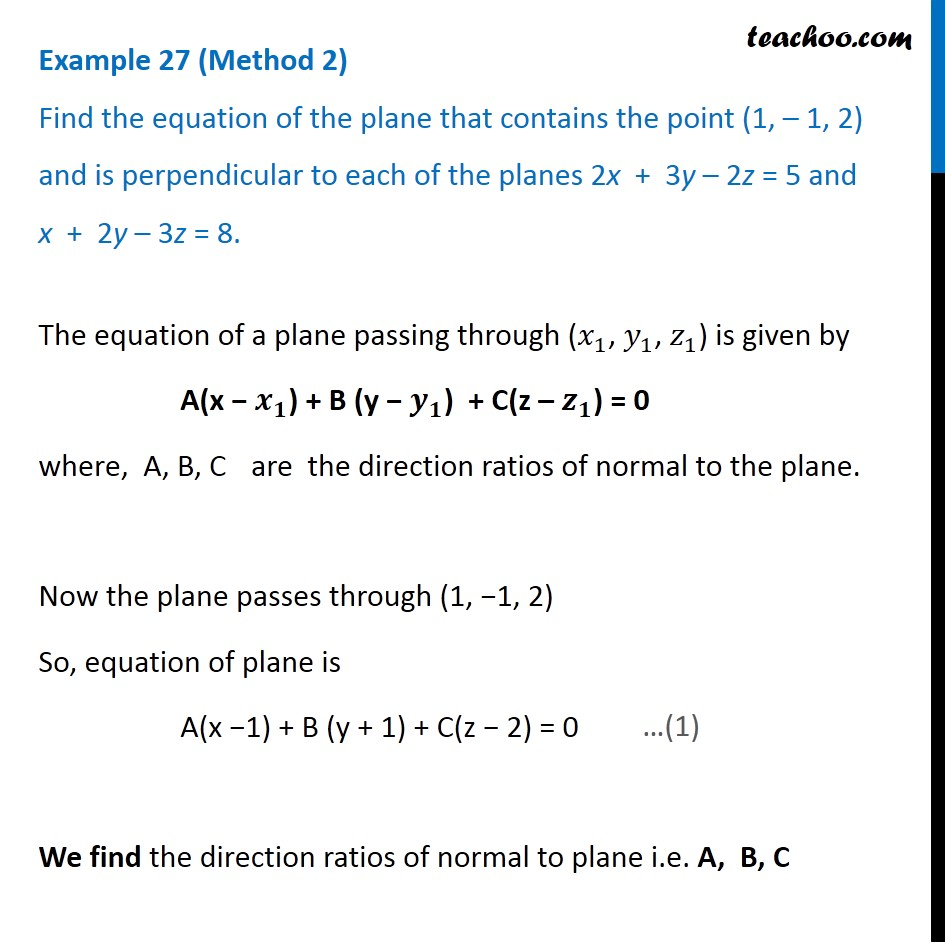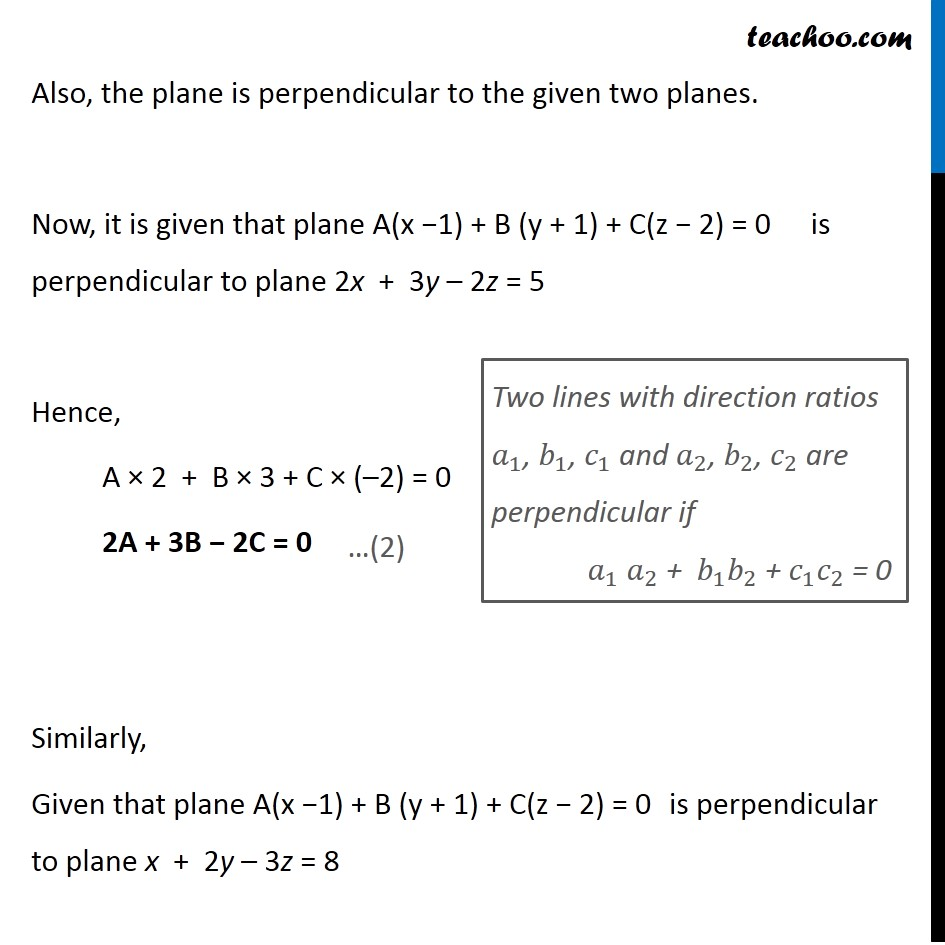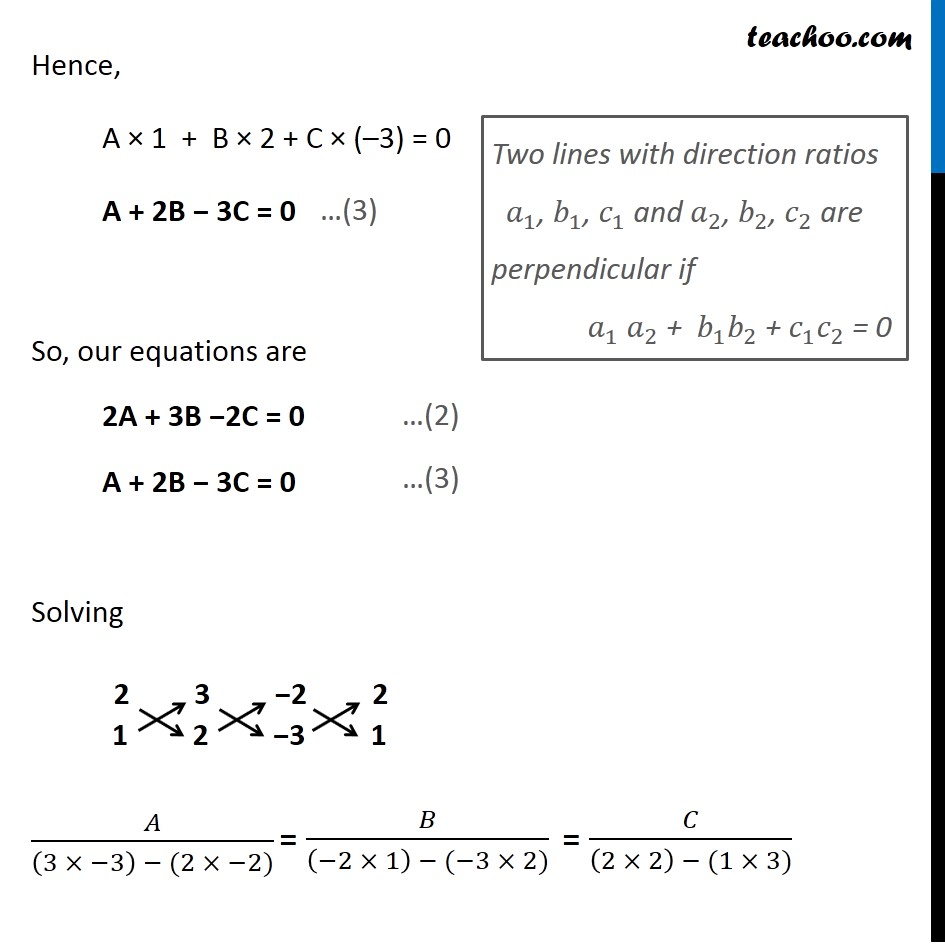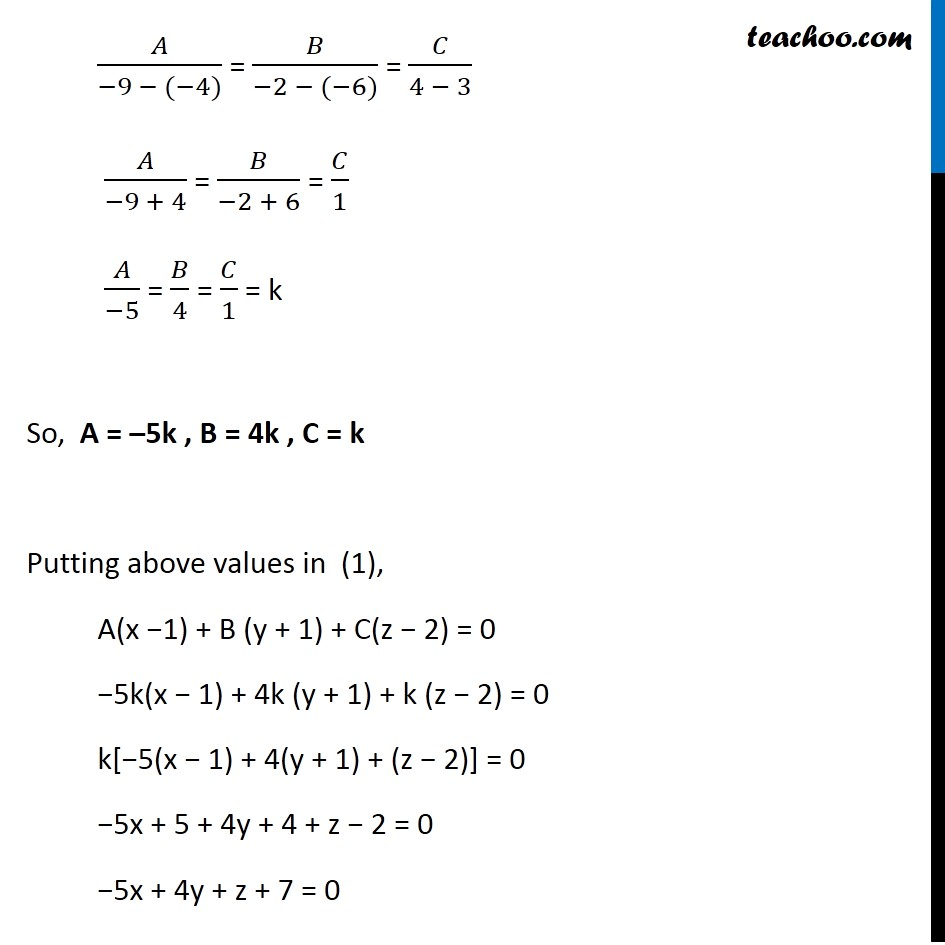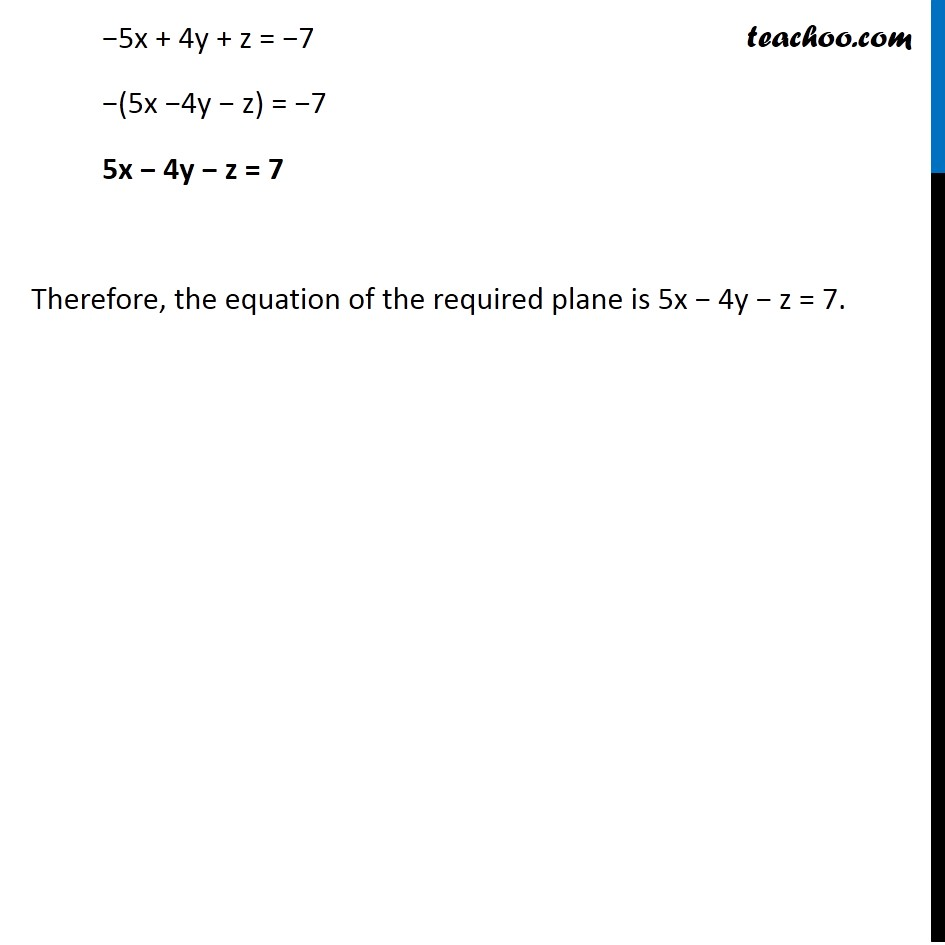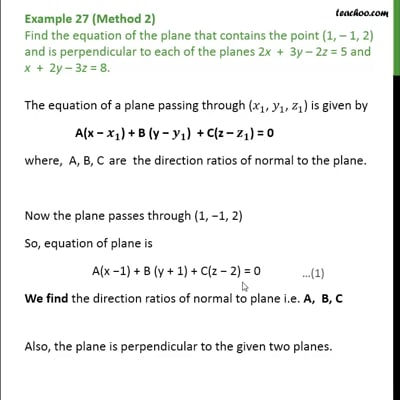This video is only available for Teachoo black users

Learn in your speed, with individual attention - Teachoo Maths 1-on-1 Class

### Transcript

Question 17 (Method 1) Find the equation of the plane that contains the point (1, –1, 2) and is perpendicular to each of the planes 2x + 3y – 2z = 5 and x + 2y – 3z = 8. The equation of a plane passing through (𝑥_1, 𝑦_1, 𝑧_1) is given by A(x − 𝒙_𝟏) + B (y − 𝒚_𝟏) + C(z – 𝒛_𝟏) = 0 where, A, B, C are the direction ratios of normal to the plane. Now the plane passes through (1, −1, 2) So, equation of plane is A(x −1) + B (y + 1) + C(z − 2) = 0 We find the direction ratios of normal to plane i.e. A, B, C Also, the plane is perpendicular to the given two planes, So, their normal to plane would be perpendicular to normal of both planes. We know that 𝑎 ⃗ × 𝑏 ⃗ is perpendicular to both 𝑎 ⃗ & 𝑏 ⃗ So, required is normal is cross product of normal of planes 2x + 3y – 2z = 5 and x + 2y – 3z = 8. Required normal = |■8(𝑖 ̂&𝑗 ̂&𝑘 ̂@2&3&−2@1&2&−3)| = 𝑖 ̂ (3(–3) – 2(–2)) – 𝑗 ̂ (2(–3) – 1(–2)) + 𝑘 ̂(2(2) – 1(3)) = 𝑖 ̂ (–9 + 4) – 𝑗 ̂ (–6 + 2) + 𝑘 ̂(4 – 3) = –5𝑖 ̂ + 4𝑗 ̂ + 𝑘 ̂ Hence, direction ratios = –5, 4, 1 ∴ A = –5, B = 4, C = 1 Putting above values in (1), A(x −1) + B (y + 1) + C(z − 2) = 0 −5(x − 1) + 4 (y + 1) + 1 (z − 2) = 0 −5x + 5 + 4y + 4 + z − 2 = 0 −5x + 4y + z + 7 = 0 −5x + 4y + z = −7 −(5x −4y − z) = −7 5x − 4y − z = 7 Therefore, the equation of the required plane is 5x − 4y − z = 7. Question 17 (Method 2) Find the equation of the plane that contains the point (1, – 1, 2) and is perpendicular to each of the planes 2x + 3y – 2z = 5 and x + 2y – 3z = 8. The equation of a plane passing through (𝑥_1, 𝑦_1, 𝑧_1) is given by A(x − 𝒙_𝟏) + B (y − 𝒚_𝟏) + C(z – 𝒛_𝟏) = 0 where, A, B, C are the direction ratios of normal to the plane. Now the plane passes through (1, −1, 2) So, equation of plane is A(x −1) + B (y + 1) + C(z − 2) = 0 We find the direction ratios of normal to plane i.e. A, B, C Also, the plane is perpendicular to the given two planes. Now, it is given that plane A(x −1) + B (y + 1) + C(z − 2) = 0 is perpendicular to plane 2x + 3y – 2z = 5 Hence, A × 2 + B × 3 + C × (–2) = 0 2A + 3B − 2C = 0 Similarly, Given that plane A(x −1) + B (y + 1) + C(z − 2) = 0 is perpendicular to plane x + 2y – 3z = 8 Two lines with direction ratios 𝑎_1, 𝑏_1, 𝑐_1 and 𝑎_2, 𝑏_2, 𝑐_2 are perpendicular if 𝑎_1 𝑎_2 + 𝑏_1 𝑏_2 + 𝑐_1 𝑐_2 = 0 Hence, A × 1 + B × 2 + C × (–3) = 0 A + 2B − 3C = 0 So, our equations are 2A + 3B −2C = 0 A + 2B − 3C = 0 Solving Two lines with direction ratios 𝑎_1, 𝑏_1, 𝑐_1 and 𝑎_2, 𝑏_2, 𝑐_2 are perpendicular if 𝑎_1 𝑎_2 + 𝑏_1 𝑏_2 + 𝑐_1 𝑐_2 = 0 𝐴/(−9 − (−4)) = 𝐵/(−2 − (−6)) = 𝐶/(4 − 3) 𝐴/(−9 + 4) = 𝐵/(−2 + 6) = 𝐶/1 𝐴/(−5) = 𝐵/4 = 𝐶/1 = k So, A = –5k , B = 4k , C = k Putting above values in (1), A(x −1) + B (y + 1) + C(z − 2) = 0 −5k(x − 1) + 4k (y + 1) + k (z − 2) = 0 k[−5(x − 1) + 4(y + 1) + (z − 2)] = 0 −5x + 5 + 4y + 4 + z − 2 = 0 −5x + 4y + z + 7 = 0 −5x + 4y + z = −7 −(5x −4y − z) = −7 5x − 4y − z = 7 Therefore, the equation of the required plane is 5x − 4y − z = 7.